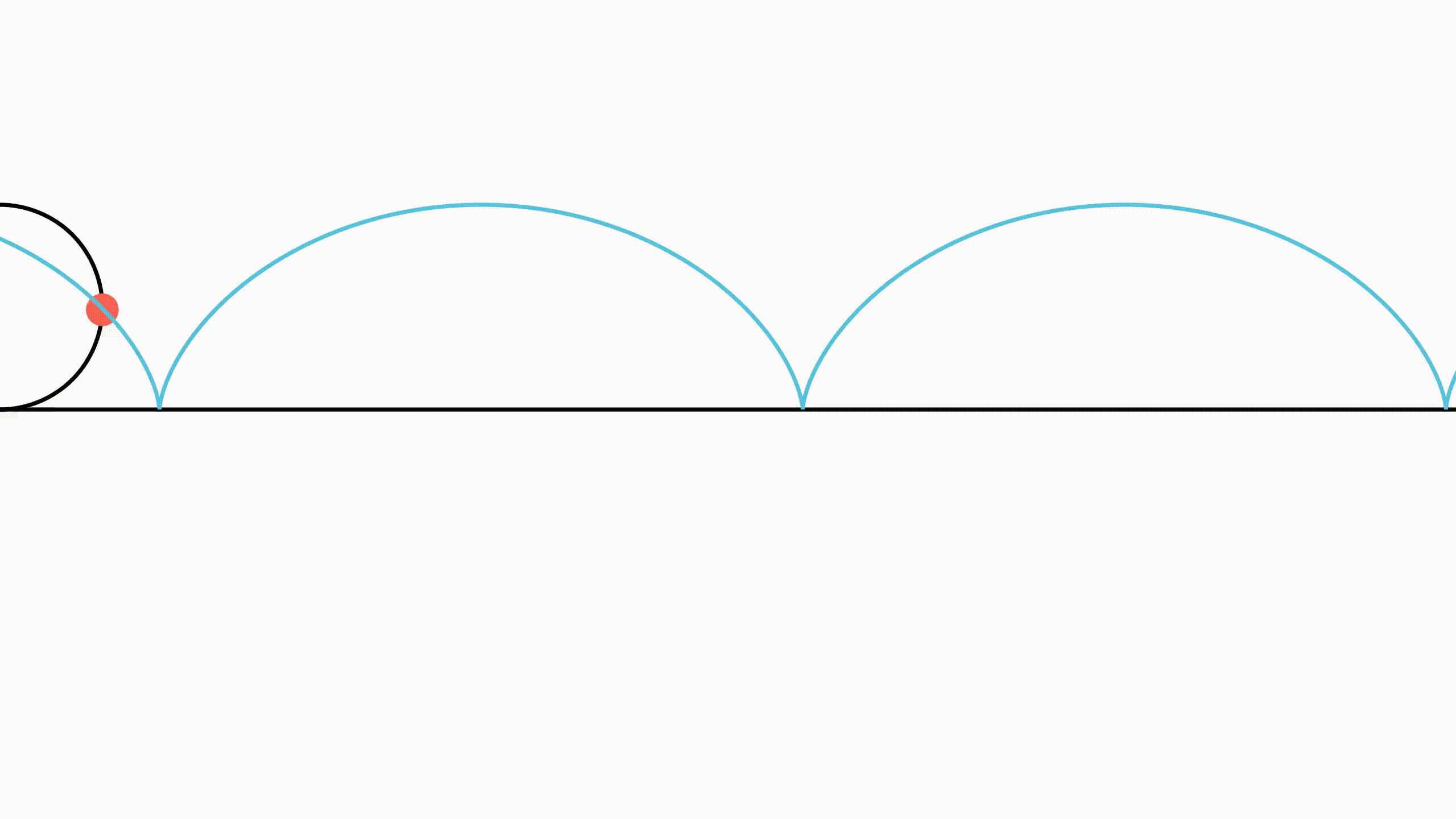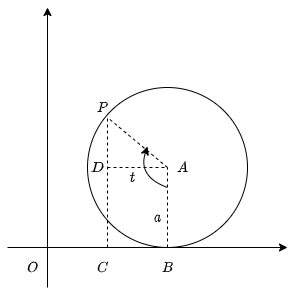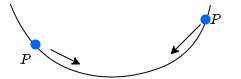# General Parametric Curves

In this post, we will take a look at some of the general parametric curves in $\mathbb{R}^{2}$ as well as $\mathbb{R}^{3}$, viz., circles, cycloids, and helixes; explore their curves to see how and why their respective equations describe such paths.

# Circles

A circle can be defined as the locus of all points that satisfy the equations $$x = a\cos{\theta}\quad y = a\sin{\theta}$$ where $x$, $y$ are the coordinates of any point on the circle, $a$ is the radius of the circle and $\theta$ is the parameter - the angle subtended by the point at the centre of the circle.

0

0

Figure 1: Circle as a parametric curve

Another way to parameterize a circle by rational functions is: $$x = \frac{1-\theta^{2}}{1+\theta^{2}}\quad y = \frac{2\theta}{1+\theta^{2}}$$

It is implied that any point not on the circle does not satisfy this pair of equations.

If the centre of the circle does not lie on the origin, we translate the circle from the origin to its proper location, i.e.:$$x = h + a\cos{\theta}\quad y = k + a\sin{\theta}$$ where $(h,k)$ is the centre of the circle.

# Cycloids

Let $P$ be a fixed point on a circle. If the circle rolls along a straight line with uniform velocity and without slipping, the path traced out by $P$ is called a cycloid

0

0Figure 2: Cycloid

The parametric equations of a cycloid are given by:$$x = at - a\sin{t}\quad y = a - a\cos{t}$$ Let's attempt to understand intuitively how these equations describe the path of a cycloid. Closely observe the motion of the red point on the circle, $P$. The x-coordinate of this point, apart from describing the x-coordinate of a circle also has to move ahead uniformly with time. The y-coordinate of this point oscillates between 0 and $a$, the radius of the circle.

Let us formally derive these parametric equations, based on these observations. Consider the situation when the circle has rolled away from the origin. Let $a$ be the radius and $t$ be the angle made by the point $P$, the centre of the circle $A$, and $B$ the point of contact of the circle with the x-axis.

0

0Figure 3: Derivation of parametric equations of cycloid

The $x$ - coordinate of $P$ is $\left|OC\right| = \left|OB\right| - \left|BC\right|$. Notice how the length the circle has rolled along the x-axis $(\left|OB\right|)$ is equal to the length of the arc $BP$. $\implies \left|OB\right| = at$.

Notice that $\angle DAP = t − \pi /2$. Hence, $\cos{(\angle DAP)} = \cos{(t - \pi /2)} = \sin{t}$. However, from the right triangle DAP, $\cos{(\angle DAP)} = \frac{AD}{a}$. Finally, $BC = AD = a\sin{t}$.

$P = (\left|OC\right|,\left|PC\right|)$

Hence, the parametric equations of a cycloid are given by:$$x = at - a\sin{t}\quad y = a - a\cos{t}$$

The cycloid provides the solution to two famous problems in mathematics:

• Brachistochrone Problem: Find the curve along which a moving particle slides under the influence of gravity, from one point to another, not directly beneath the first point, in the shortest time.

0

0Figure 4: Brachistochrone problem

• Tautochrone Problem: Find the curve having the property that it takes the same time for a particle to slide to the bottom of the curve no matter where the particle is placed on the curve.

0

0Figure 5: Tautochrone problem

# Helixes

A helix is defined as a curve for which the tangent makes a constant angle with a fixed line. Parametrically, the equation of a helix are given by: $$\overrightarrow{r}(t) = \left\langle a\cos{t}, a\sin{t}, \lambda t \right\rangle$$

It is a noteworthy fact that the above curve must lie on the circular cylinder $x^2 + y^2 = a^2$.

As $t$ increases from $0$ to $2n\pi$, the point $(x, y, z)$ moves in an upward clockwise cylinder creating a circular curve called the helix.

0

0

Figure 6: Visualization of a helix

Also note that the difference between $\left\langle a\cos{t}, a\sin{t}, \lambda t \right\rangle$, $\left\langle \lambda t, a\cos{t}, a\sin{t}\right\rangle$ and $\left\langle a\cos{t}, \lambda t, a\sin{t}\right\rangle$ is the direction of propogation of the helix, i.e., $z$-axis, $x$-axis, and $y$-axis respectively.

# Pause and Ponder

• Can a curve parameterized in time be considered to have a physical reality?

# References

 https://physics.info/parametric/

### Images

 Figure 3 has been generated using draw.io

0

 Contributor: Mentor & Editor: Verified by: Approved On:

The following notes and their corrosponding animations were created by the above-mentioned contributor and are freely avilable under CC (by SA) licence. The source code for the said animations is avilable on GitHub and is licenced under the MIT licence.

The work under this website is licenced under a Creative Commons Attribution-Share Alike 4.0 International License CC BY-SA